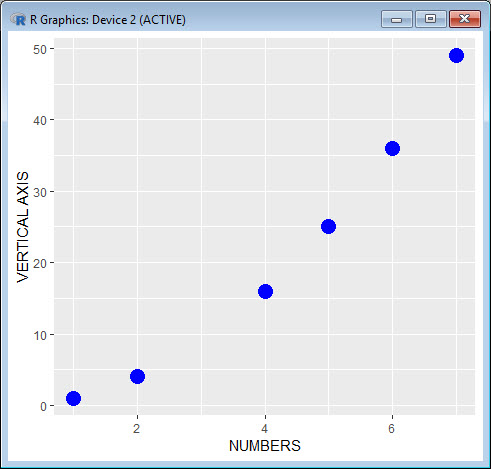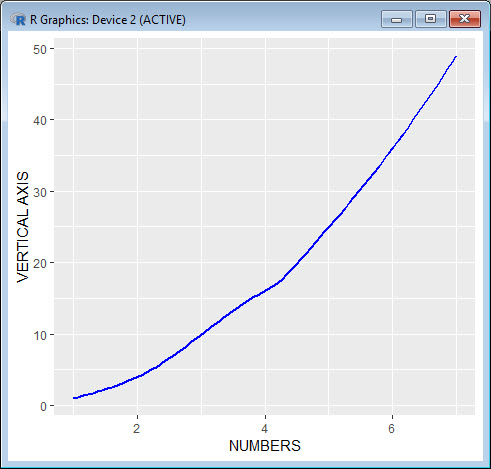In Part 23, let’s see how to use `qplot` to create a simple scatterplot.
The `qplot` (quick plot) system is a subset of the `ggplot2` (grammar of graphics) package which you can use to create nice graphs. It is great for creating graphs of categorical data, because you can map symbol colour, size and shape to the levels of your categorical variable. To use `qplot` first install `ggplot2` as follows:
`install.packages("ggplot2")`
and then load `ggplot2` using the command:
`library(ggplot2)`
The `qplot` syntax is as follows:
`qplot(x = X, y = X, data = X, colour = X, shape = X, geom = X, main = "Title")`
where `x` gives the `x` values you wish to plot.
`y` gives the `y` values you wish to plot. You now have bivariate data and must provide an appropriate `geom`.
`data` gives the object name of the data frame.
`colour` maps the colour scheme onto a factor variable, and `qplot` now selects different colours for different levels of the variable. You can use special syntax to set your own colours.
`shape` maps the symbol shapes onto a factor variable, and `qplot` now selects different shapes for different levels of the factor variable. You can use special syntax to set your own shapes.
`geom` provides a list of keywords that control the kind of plot, including: “`histogram`“, “`density`“, “`line`“, “`point`“.
`main` provides the title for the plot.
In `qplot`, you can set your desired aesthetics using the operator `I()`. For example, if you want red use: `color = I("red")`. If you want to control the size of the symbols, use: `size = I(N)`, where a value of `N` greater than 1 expands the symbols. For example, `size = I(5)` produces very big symbols.
Anyway – let’s start with a simple example where we set up a simple scatterplot with blue symbols. Now read in this dataset:
`T <- structure(list(A = c(1, 2, 4, 5, 6, 7), B = c(1, 4, 16, 25, 36, 49)), .Names = c("A", "B"), row.names = c(NA, -6L), class = "data.frame")`
`T`Now plot `A` against `B` using `I()` for colour and symbol size. We include axis labels of our choice and use symbol size 5 (large symbols).
`qplot(A, B, data = T, xlab = "NUMBERS", ylab = "VERTICAL AXIS", colour = I("blue"), size = I(5))`Note the default background, grey in colour and including a grid. We can modify those attributes quite easily and we will do so in a later blog
Now we create a scatterplot with a smooth curve using `geom = c("smooth")`.
`qplot(A, B, data = T, xlab = "NUMBERS", ylab = "VERTICAL AXIS", colour = I("blue"), size = I(1), geom = c("smooth"))`We chose `size = I(1)` for this example, but we can include a larger value to get a thicker line.
That wasn’t so hard! In Blog 24 we will look at further plotting techniques using `qplot`.
See you later!
David

#### Annex: R codes used

```# Install ggplot2 package.
install.packages("ggplot2")